# Python For Loop

## Introduction

In this chapter, we will discuss Python's for a loop. Same as while loop, for loop, also control flow statement. In Python, for loop is used when we want to iterate over a sequence.

## Python For Loop

Syntax
1. for IterationalVariable in sequence:
2.         Body of the loop
In Python, for loop works like C# foreach loop.

### For loop with a list

The following example explains how can you iterate a list using for loop.

Example
1. list=['C','C++','Java','C#']
2. print("This is our list ",list)
3. i=1
4. for val in list:
5.     print("List Item",i,"is",val)
6.     i=i+1
Output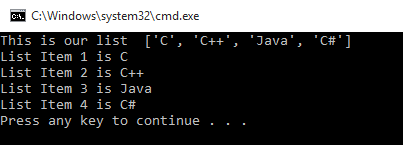### For loop with tuple

The following example explains how can you iterate tuple using for loop.

Example
1. tuple=('C','C++','Java','C#','Python')
2. print("This is our Tuple ",tuple)
3. i=1
4. for val in tuple:
5.     print("Tuple Item",i,"is",val)
6.     i=i+1
Output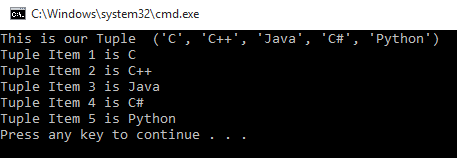### For loop with string

The following example explains how can you iterate string using for loop.

Example
1. string="C-Sharp Corner"
2. for val in string:
3.     print(val)
Output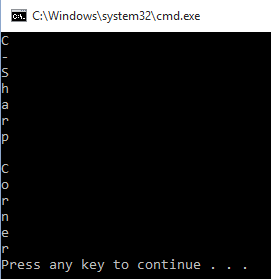## Range Function

Sometimes we need to iterate loop in a given range and we cannot create a sequence. For example, if you want to print a number from one to 1000, so will you create a sequence or list from 1 to 1000? Of course, you cannot go like this. So in Python, we have a range function, in which we will decide range inside the function.

Syntax of range function
1. range([start],end,[step])
In Python range function has 3 arguments In which two are optional, which are the following:
• start- This is an optional argument. This argument decides from where you want to start your loop. If you do not provide this value then its value will be zero(0) by default.

• end- This argument decides the end of the loop.

• step- How much you want to increment after one iteration. This is also an optional argument. So if you do not give this argument then it will increment by 1 step.
Example 1
1. for val in range(11):
2.     print(val)
The above example of the range contains only one parameter so that it will be the end parameter and start and step will be set with its default value, so start will be 0 and the step will be 1 and the value of end is 11.

Output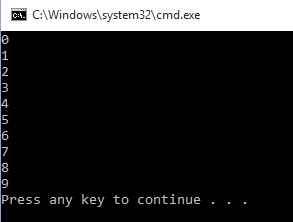Example 2
1. for val in range(1,11):
2.     print(val)
The above example of the range contains two parameters so that it will be the start and end parameter and the step will be set with its default value. So in the above example. start=1,end=11 and step=1(default).

Output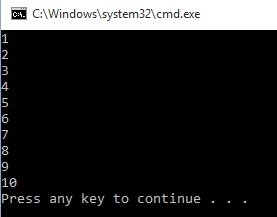Example 3
1. for val in range(1,11,2):
2.     print(val)
In the above example start=1 end=11 and step=2.

Output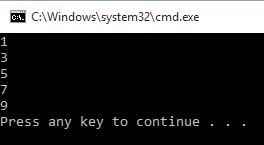### For loop with else condition

Same as while loop you can also use for loop with else condition in python. For loop works the same as normal for loop but whenever for loop condition will be false it's else part will execute.

Note

else part will only execute when for loop condition will be false. If for loop will be terminated by break statement then for loop's else part never executes.

Example
1. #program to check
2. #weather the given number is
3. #prime number or not
4. num = int(input("Enter a Number : "))
5. for i in range(2,num):
6.     if(num%i==0):
7.         print("%d is not a prime number..."%num)
8.         break
9. else:
10.     print("%d is a prime number..."%num)
Output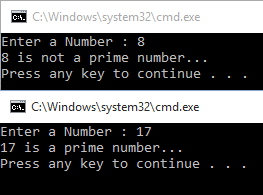## Nested Loop

A nested loop means a loop inside the loop. In python, you can create a loop inside a loop.

Example 1
1. str="";
2. for Row in range(0,7):
3.     for Col in range(0,7):
4.         if (((Col == 1 or Col == 5and Row != 0or ((Row == 0 or Row == 3and (Col > 1 and Col < 5))):
5.             str=str+"*"
6.         else:
7.             str=str+" "
8.     str=str+"\n"
9. print(str);
Output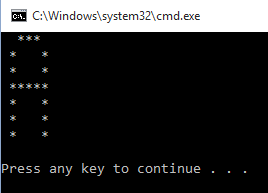Example 2
1. str="";
2. for Row in range(0,7):
3.     for Col in range(0,7):
4.         if (Col == 1 or ((Row == 0 or Row == 3 or Row == 6and (Col < 5 and Col > 1)) or (Col == 5 and (Row != 0 and Row != 3 and Row != 6)))  :
5.             str=str+"*"
6.         else:
7.             str=str+" "
8.     str=str+"\n"
9. print(str);
Output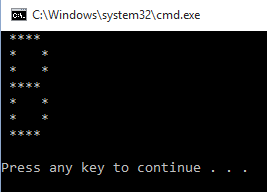Example 3
1. row=15
2. col=18
3. str=""
4. for i in range(1,row+1):
5.     if((i<=3)or(i>=7 and i<=9)or(i>=13 and i<=15)):
6.         for j in range(1,col):
7.             str=str+"*"
8.         str=str+"\n"
9.     elif(i>=4 and i<=6):
10.         for j in range(1,5):
11.             str=str+"*"
12.         str=str+"\n"
13.     else:
14.         for j in range(1,14):
15.             str=str+" "
16.         for j in range(1,5):
17.             str=str+"*"
18.         str=str+"\n"
19. print(str)
Output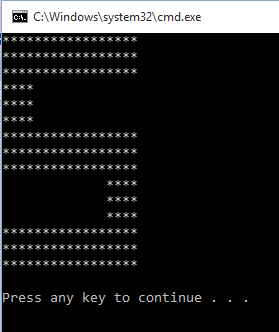## Summary

In the next chapter, you will learn how to use Python functions.
Next » Python Functions